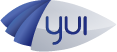#API Docs for: 3.5.1
Show:

# CanvasDrawing Class

Canvas implementation of the `Drawing` class. `CanvasDrawing` is not intended to be used directly. Instead, use the `Drawing` class. If the browser lacks SVG capabilities but has Canvas capabilities, the `Drawing` class will point to the `CanvasDrawing` class.

## Methods

### `_clearAndUpdateCoords`

() private

Clears the coordinate arrays. Called at the end of a drawing operation.

### `_createGraphic`

() private

Creates canvas element

#### Returns:

HTMLCanvasElement

() private

() private

### `_initProps`

() private

Clears all values

### `_toRGB`

(
• `val`
)
private

Converts color to rgb format

#### Parameters:

• `val` Object

Color value to convert.

### `_toRGBA`

(
• `val`
• `alpha`
)
private

Parses hex color string and alpha value to rgba

#### Parameters:

• `val` Object

Color value to parse. Can be hex string, rgb or name.

• `alpha` Number

Numeric value between 0 and 1 representing the alpha level.

### `_trackSize`

(
• `w`
• `h`
)
private

Updates the size of the graphics object

#### Parameters:

• `w` Number

width

• `h` Number

height

### `_updateCoords`

(
• `x`
• `y`
)
private

Tracks coordinates. Used to calculate the start point of dashed lines.

#### Parameters:

• `x` Number

x-coordinate

• `y` Number

y-coordinate

### `_updateDrawingQueue`

(
• `val`
)
private

Queues up a method to be executed when a shape redraws.

#### Parameters:

• `val` Array

An array containing data that can be parsed into a method and arguments. The value at zero-index of the array is a string reference of the drawing method that will be called. All subsequent indices are argument for that method. For example, `lineTo(10, 100)` would be structured as: `["lineTo", 10, 100]`.

### `_updateNodePosition`

() private

Moves the shape's dom node.

### `clear`

()

Clears the graphics object.

### `closePath`

()

Ends a fill and stroke

### `curveTo`

(
• `cp1x`
• `cp1y`
• `cp2x`
• `cp2y`
• `x`
• `y`
)

Draws a bezier curve.

#### Parameters:

• `cp1x` Number

x-coordinate for the first control point.

• `cp1y` Number

y-coordinate for the first control point.

• `cp2x` Number

x-coordinate for the second control point.

• `cp2y` Number

y-coordinate for the second control point.

• `x` Number

x-coordinate for the end point.

• `y` Number

y-coordinate for the end point.

### `drawCircle`

(
• `x`
• `y`
• `r`
)
protected

Draws a circle. Used internally by `CanvasCircle` class.

#### Parameters:

• `x` Number

y-coordinate

• `y` Number

x-coordinate

• `r` Number

### `drawDiamond`

(
• `x`
• `y`
• `width`
• `height`
)
protected

Draws a diamond.

#### Parameters:

• `x` Number

y-coordinate

• `y` Number

x-coordinate

• `width` Number

width

• `height` Number

height

### `drawEllipse`

(
• `x`
• `y`
• `w`
• `h`
)
protected

Draws an ellipse. Used internally by `CanvasEllipse` class.

#### Parameters:

• `x` Number

x-coordinate

• `y` Number

y-coordinate

• `w` Number

width

• `h` Number

height

### `drawRect`

(
• `x`
• `y`
• `w`
• `h`
• `ew`
• `eh`
)

Draws a rectangle with rounded corners.

#### Parameters:

• `x` Number

x-coordinate

• `y` Number

y-coordinate

• `w` Number

width

• `h` Number

height

• `ew` Number

width of the ellipse used to draw the rounded corners

• `eh` Number

height of the ellipse used to draw the rounded corners

### `drawRect`

(
• `x`
• `y`
• `w`
• `h`
)

Draws a rectangle.

#### Parameters:

• `x` Number

x-coordinate

• `y` Number

y-coordinate

• `w` Number

width

• `h` Number

height

### `drawWedge`

(
• `x`
• `y`
• `startAngle`
• `arc`
• `radius`
• `yRadius`
)
private

Draws a wedge.

#### Parameters:

• `x` Number

x-coordinate of the wedge's center point

• `y` Number

y-coordinate of the wedge's center point

• `startAngle` Number

starting angle in degrees

• `arc` Number

sweep of the wedge. Negative values draw clockwise.

• `radius` Number

• `yRadius` Number

### `end`

()

Completes a drawing operation.

### `lineTo`

(
• `point1`
• `point2`
)

Draws a line segment using the current line style from the current drawing position to the specified x and y coordinates.

#### Parameters:

• `point1` Number

x-coordinate for the end point.

• `point2` Number

y-coordinate for the end point.

### `moveTo`

(
• `x`
• `y`
)

Moves the current drawing position to specified x and y coordinates.

#### Parameters:

• `x` Number

x-coordinate for the end point.

• `y` Number

y-coordinate for the end point.

### `quadraticCurveTo`

(
• `cpx`
• `cpy`
• `x`
• `y`
)

#### Parameters:

• `cpx` Number

x-coordinate for the control point.

• `cpy` Number

y-coordinate for the control point.

• `x` Number

x-coordinate for the end point.

• `y` Number

y-coordinate for the end point.

### `setSize`

(
• `w`
• `h`
)
private

Sets the size of the graphics object.

#### Parameters:

• `w` Number

width to set for the instance.

• `h` Number

height to set for the instance.

## Properties

### `_drawingComplete`

Boolean private

Indicates a drawing has completed.